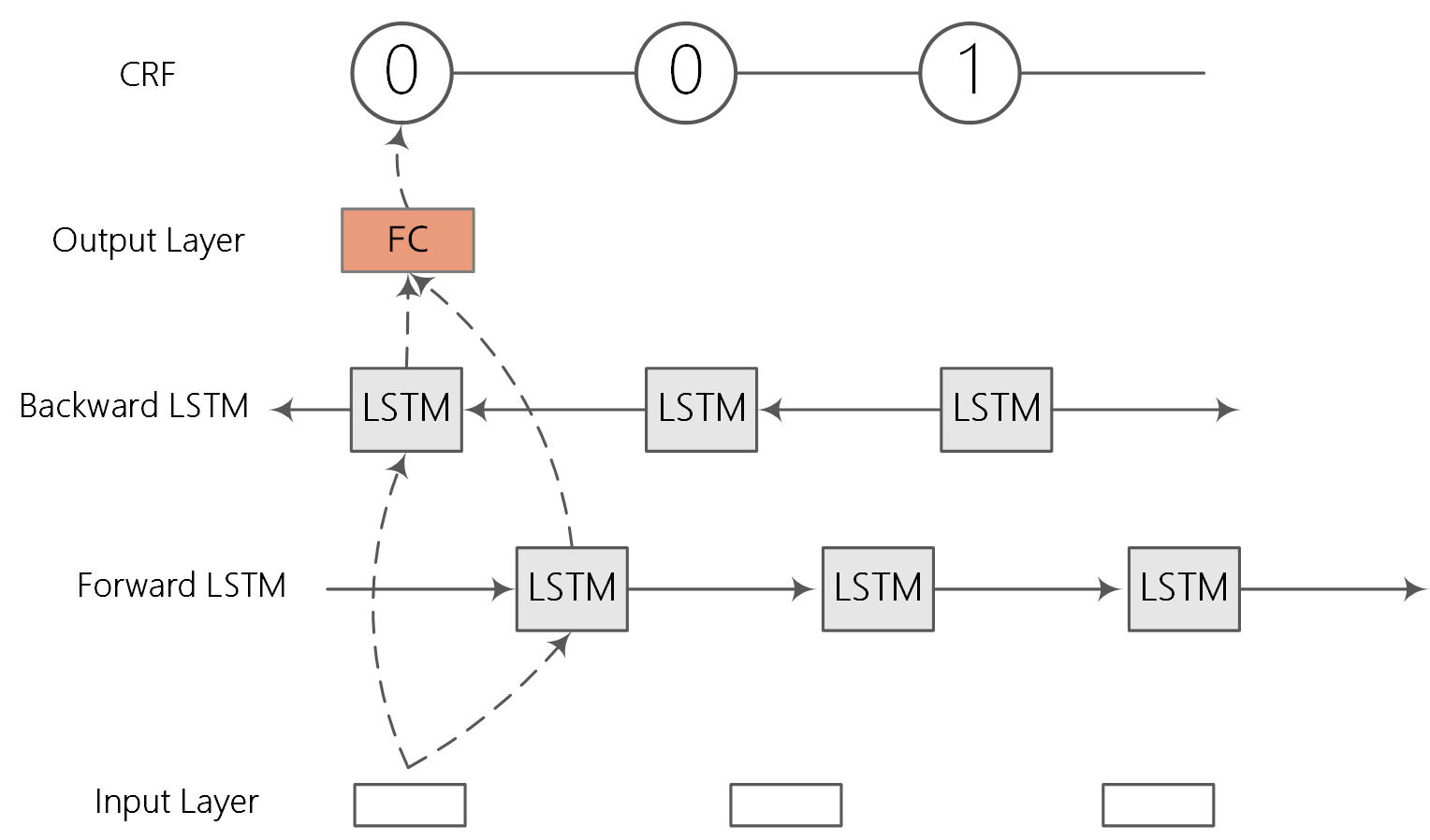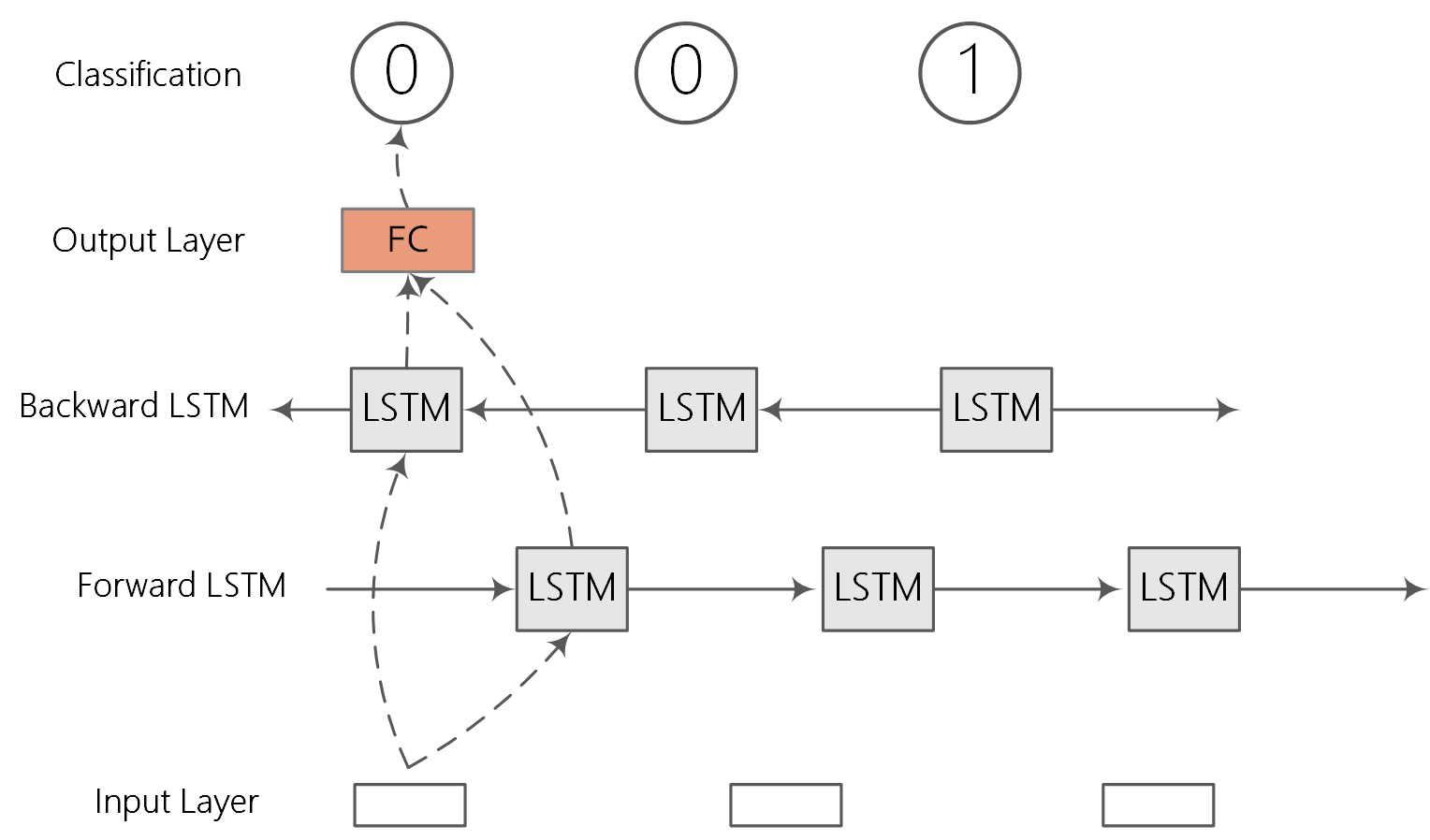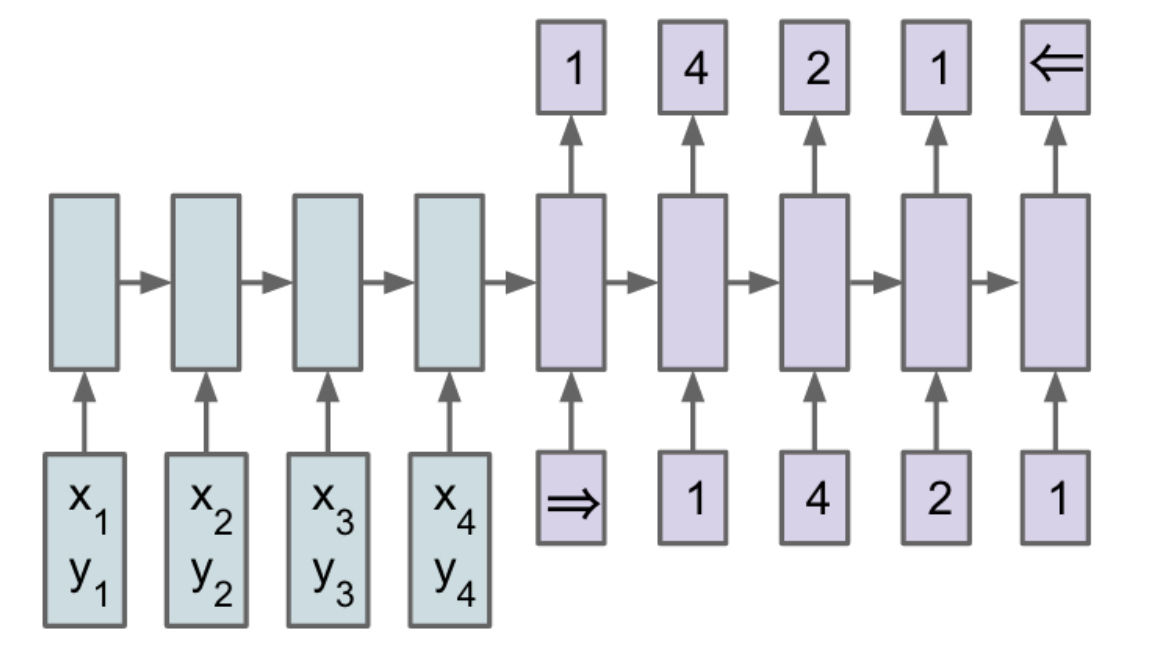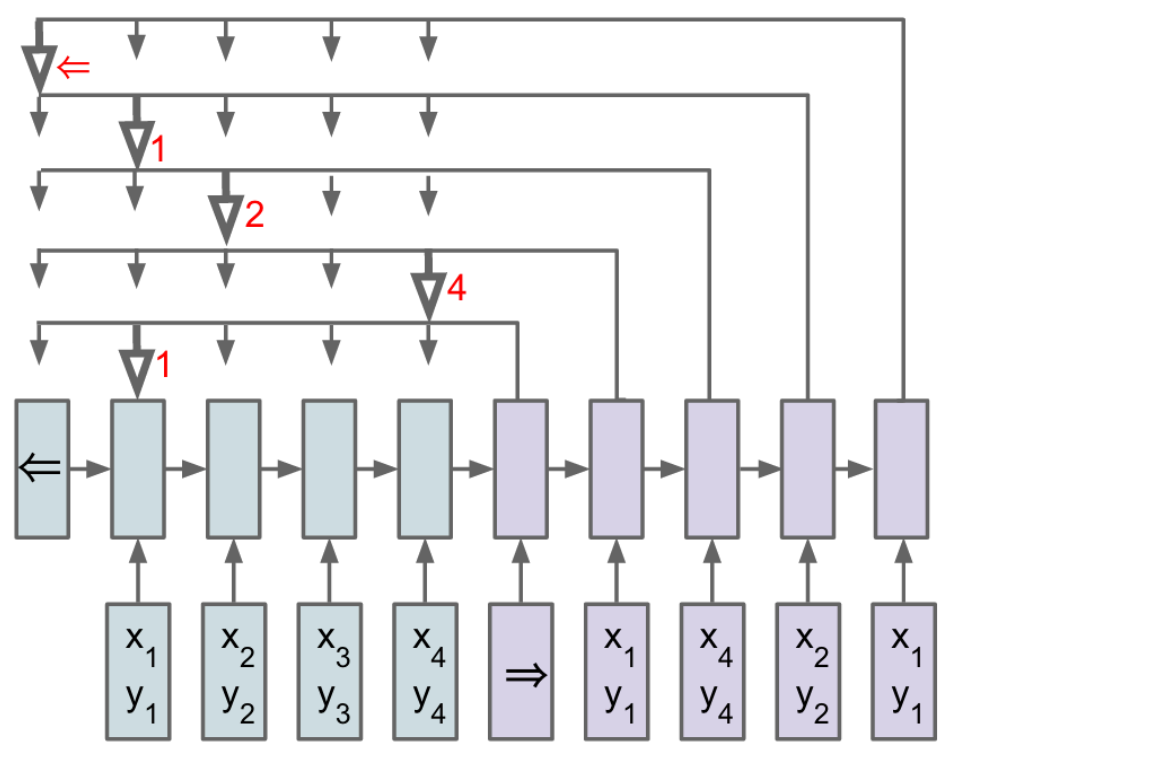Oct 7, 2017 by Li Jing

This post explains the maths behind sequence labeling using HMMs, MEMMs, CRFs, Naive classification, seq2seq, Pointer-network.

Sequence labeling problem:
Input sequence: x_1,x_2,...,x_m
Output sequence: y_1,y_2,...,y_m

1. Hidden Markov Models (HMMs)
2. The task is to model the joint probability: $\require{color} \colorbox{yellow}{ p(y_1,y_2,...,y_m, x_1,x_2,...,x_m) }= \prod\limits_{i = 1}^m p(y_i|y_{i-1}) \prod\limits_{i = 1}^m {p(x_i|y_i)}$ Independence Assumption: Consider two random Variables: X andY, the task will be to model the joint probability: $P(Y_1=y_1,...,Y_n=y_n, X_1=x_1...X_n=x_n) = P(Y_1=y_1,...,Y_n=y_n) P(X_1=x_1...X_n=x_n|Y_1=y_1,...,Y_n=y_n)$ This step is exact, by the chian rule of probabilities.
Assumption 1: $P(Y_1=y_1,...,Y_n=y_n) \approx \prod\limits_{i = 1}^m {P(Y_i=y_i|Y_{i-1}=y_{i-1})}$ Assumption 2: \begin{align} P(X_1=x_1...X_n=x_n|Y_1=y_1,...,Y_n=y_n) &= \prod\limits_{i = 1}^m {P(X_i=x_i|X_1=x_1...X_{i-1}=x_{i-1},Y_1=y_1...Y_n=y_n)} \\ &\approx \prod\limits_{i = 1}^m {P(X_i=x_i|Y_i=y_i)} \end{align} That is the value for random variable X_i depends only on the value of Y_i. More formally, the value for X_i in conditionally independent of the previous observations X_1...X_{i-1} and the other state values Y_1,...Y_{i-1},Y_{i+1},...Y_n, given the value of Y_i.
Estimating the parameters of the model is simple: we just read off counts from the training corpus, and then compute the maximum-likelihood estimates as described above.

3. Maximum-entropy Markov Models (MEMMs)
4. The task will be to model the conditional distribution \begin{align} p(y_1,y_2,...,y_m|x_1,x_2,...,x_m) &= \prod\limits_{i = 1}^m {p(y_i|y_1,...,y_{i-1},x_1,...,x_m)} \\ & \approx \prod\limits_{i = 1}^m {p(y_i|y_{i-1},x_1,...,x_m)} \end{align} Assumption: The state y_i depends only on the state y_{i-1}.

The key advantage of MEMMs is that the use of feature vectors $$\boldsymbol{F} \in \mathbb{R}^d$$ allows much richer representation than those used in HMMs.

Base on the assumption, we model each term using a log-linear model:

$\require{color} \colorbox{yellow}{p(y_i|y_{i-1},x_1,...,x_m) } = \frac{\exp \left(\boldsymbol{w} \cdot \boldsymbol{F}(x_1,...x_m,i,y_{i-1},y_i) \right )} {\sum\nolimits_{\color{red} {y' \in \mathcal{S}}} \exp \left(\boldsymbol{w} \cdot \boldsymbol{F}(x_1,...x_m,i,y_{i-1},y') \right ) }$ where $$\boldsymbol{F}(x_1,...x_m,i,y_{i-1},y_i)$$ is a feacture vector. $$\mathcal{S}$$ denotes the set of possible states. $$\boldsymbol{w}$$ are the parameters.

5. Conditional Random Fields (CRFs)
6.Figure: BiLSTM-CRF.
The task will be to model the conditional distribution $p(y_1,y_2,...,y_m|x_1,x_2,...,x_m) = p(\vec y| \vec x)$

First, we define a feature vector $$\boldsymbol{F}(\vec x,\vec y) \in \mathbb{R}^d$$. CRF key idea: $$\boldsymbol{F}(\vec x,\vec y)$$ maps an entire input sequence $$\vec x$$ paired with an entire state sequence $$\vec y$$ to some $$d$$-dimensional feature vectors. $$\boldsymbol{F}(\vec x,\vec y)$$ is a "global" feature vector (it is global in the sense that it takes the entire state sequence into account).

$\boldsymbol{F}(\vec x,\vec y) = \sum\limits_i^m f(\vec x, i, y_{i-1},y_i)$ We are assuming that $$k=1,...,d$$, the k-th global feature is: $\boldsymbol{F}_k(\vec x,\vec y) = \sum\limits_i^m f_k(\vec x, i, y_{i-1},y_i)$ We can see that $$\boldsymbol{F}_k$$ is calculated by summing the "local" feature vector f_k over the m different state transitions in y_1,...,y_m.
We then build a log-linear model: $\require{color} \colorbox{yellow}{ p(\vec y|\vec x; \boldsymbol{w}) }= \frac{\exp \left(\boldsymbol{w} \cdot \boldsymbol{F}(\vec x, \vec y) \right )} {\sum\nolimits_{\color{red} {\vec{y'} \in \mathcal{S}^m}} \exp \left(\boldsymbol{w} \cdot \boldsymbol{F}(\vec x, \vec {y'}) \right ) }$ We can find that the space of possible values for $$\vec{y'}$$ is $$\mathcal{S}^m$$. It is very huge.
Parameter Estimation in CRFs.
We have n training examples, $${(\vec {x^j}, \vec {y^j})}_{j=1}^n$$. Each instance $$\vec{x^j}$$ is an input sequence $$x_1^j,...,x_m^j$$, each $$\vec{y^j}$$ is a state sequence $$y_1^j,...,y_m^j$$. The regularized log-likelihood function is $L(\boldsymbol{w}) = \sum\limits_{j=1}^n \log p(\vec {y^j}|\vec {x^j}; \boldsymbol{w}) - \frac{\lambda}{2}||\boldsymbol{w}||^2$ The partial derivatives are $\frac{\partial}{\partial w_k} L(\boldsymbol{w}) = \sum\limits_{j=1}^n \boldsymbol{F}_k(\vec {x^j},\vec {y^j}) - \sum\limits_{j=1}^n \sum\limits_{\color{red} {\vec{y'} \in \mathcal{S}^m}} p(\vec{y'}|\vec{x^j};\boldsymbol{w}) \boldsymbol{F}_k(\vec{x^j},\vec{y'}) - \lambda w_k$

7. Naive Classification
8.Figure: sequence labeling as a classification.
$\require{color} \colorbox{yellow}{ p(y_i|\vec{x};\boldsymbol{w}) } = \frac{e^{f_{y_i}}}{{\sum_j e^{f_j}}}$ The cross-entropy between a "true" distribution p and an estimated distribution q is defined as: \begin{align} H(p,q) &= -\sum_x p(x)\log q(x) \\ &=-\sum_i \log \left( \frac{e^{f_{y_i}}}{{\sum_j e^{f_j}}} \right) \end{align} The Softmax classifier is hence minimizing the cross-entropy between the estimated class probabilities (q=\frac{e^{f_{y_i}}}{\sum_j e^{f_j}} as seen above) and the "true" distribution, which in this interpretation is the distribution where all probability mass is on the correct class (i.e. p=[0,...1,...,0] contains a single 1 at the y_i-th position.).

9. Sequence-to-Sequence (seq2seq)
10.Figure: Sequence-to-Sequence.
In traditional sequence-to-sequence model, the output dictionary size is fixed. For example, in chinese-english translation task, the english vocabulary is fixed when generation english sentences. Thus, we need to train a separate model for each fixed dictionary. Input sequence: $$x_i,...,x_n$$, output sequence: $$y_1,...,y_m$$, the task is to model the conditional probability: $p(\vec{y}|\vec{x}; \boldsymbol{w})= \prod \limits_{i=1}^m p(y_i|y_1,...,y_{i-1},\vec{x};\boldsymbol{w})$ With an RNN, each conditional probability is modeled as $\require{color} \colorbox{yellow}{ p(y_i|y_1,...,y_{i-1},\vec{x};\boldsymbol{w}) }= g(y_{i-1},\vec{s_i},\vec{c})$ where g is a nonlinear, potentially multi-layered, function that outputs the probability of y_i, and $$\vec{s_i}$$ is the hidden state of the RNN at time step i, and c is the context vector.

11. Pointer Networks
12.Figure: Pointer Networks.
The advantage of pointer networks is that the output dictionary size depends on the number of elements in the input sequence. That is, the output dictionary size is variable.
Let us define input sequence: $$x_i,...,x_n$$, output sequence: $$y_1,...,y_m$$, encoder hidden states $$e_1,...,e_n$$, and decoder hidden states $$d_1,...,d_m$$. The attention mechanism can be modeled as follows: \begin{align} u_j^i &= v^T tanh(W_1e_j+W_2d_i) \quad j \in (1,...,n) \\ \require{color} \colorbox{yellow}{ p(y_i|y_1,...,y_{i-1},\vec{x}) } &= softmax(\vec{u^i}) \\ \end{align} where softmax normalizes the vector $$\vec{u^i}$$ (of length n) to be an output distribution over the dictionary of inputs (length n).
v, W_1 and W_2 are learnable parameters of the output model.
The parameters of the model are learnt by maximizing the conditional probabilities for the training set, i.e. $\boldsymbol{w}^* = \underset{\boldsymbol{w}}{\arg\max} \sum \log p(\vec{y}|\vec{x};\boldsymbol{w})$ where the sum is over training examples. $$\boldsymbol{w}$$ is the set of the model parameters and each $$(\vec{x},\vec{y})$$ is an (input sequence, output sequence) pair from the training set.

References
 Log-Linear Models, MEMMs, and CRFs
 Pointer Networks
 Tagging with Hidden Markov Models
 Linear Classification